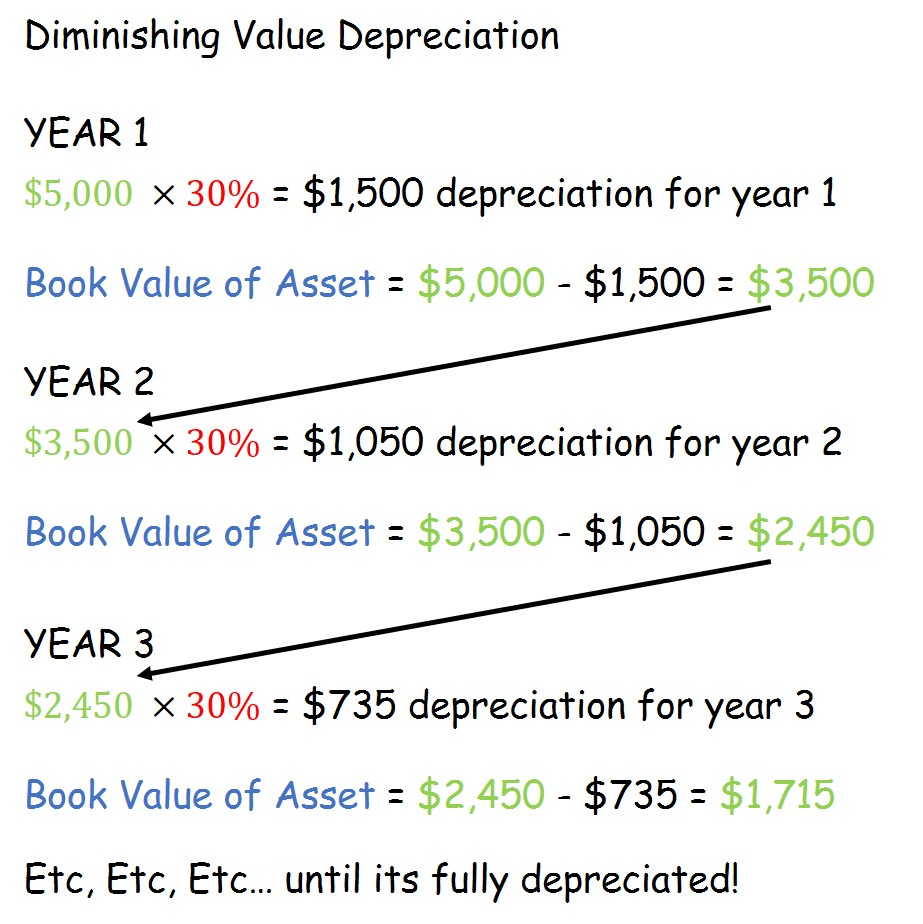### 2 simple ways to record depreciation

Depreciation!

If you are a small business owner and own any fixed assets like a car, boat, packaging machine or semi-autonomous robotic assistant, you will know that you can claim depreciation as an expense. Most of you will also know that depreciation is a representation of the wear and tear of an assets’ use over a period of time.

Essentially there are two well-accepted methods of recording depreciation:

Straight-Line depreciation

Straight line depreciation is easy. Let’s say you own a car which you bought for \$5,000. You then estimate that the car will be good for say maybe another 10 years. This means that every year, you can claim 10% of depreciation on the car every year until the end of 10 years. In simple maths, this means that you can claim \$500 of depreciation expense in your profit and loss statement.

This also means that you claim the same amount of depreciation expense on your asset every year. While this makes calculation simple, it might not reflect the true wear and tear on use of the asset.

Diminishing value depreciationThe diminishing value method is more complicated – but it makes sense from a practical usage point of view.

Diminishing value is quite different from the straight line method. Let’s take the example of that \$5,000 car again. Let’s assume its Inland Revenue approved rate is 30%. In the first year, you would claim depreciation expense of 30% of \$5,000, which is \$1,500. This amount is then deducted from the original cost amount of \$5,000 which means that there is a book value (the amount recorded on the books) of the car worth \$3,500. In the second year, depreciation expense is recorded as 30% of \$3,500 which is \$1,050. Then \$1,050 is deducted from \$3,500 leaving you with a book value of \$2,450. In the third year, your depreciation expense is 30% of \$2,450 and, well you can guess what happens next.

Every year, the book value of the asset diminishes based on the set percentage of 30% (in this case). With the diminishing value method, you get to claim a proportionately higher amount of depreciation expenses in the first year and less in the preceding years until the asset’s book value is diminished.

In the long run, you still claim the same amount of depreciation in each scenario (\$5,000) however the timing of the expenses will be different in each scenario. With the straight-line method, you distribute your depreciation expense evenly across a number of years whereas with the Diminishing value method you front-load a lot of your depreciation expenses in the first year and claim less in the preceding years.

Personally, I like the diminishing value method because from a practical point of view, I tend to get the most use out of my assets in the first few years and as it starts to wear down, I use it less in its twilight years. However there is a simplicity to the straight-line method that some people prefer. Ultimately, all accounting packages are well-equipped to handle both straight-line and diminishing value calculations so you don’t really need to worry about performing complex calculations every year when it’s time to depreciate your assets.

It is worth pointing out that the Tax Department in your country will have prescribed depreciation rates (for both Straight-line and Diminishing Value) for different types of fixed assets. Most countries will have this on their Tax Website. In NZ you can check out the IRD’s depreciation calculator right here.